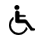Pristupačnost

Poll
Repository
Repository is empty
News
Mathematical Foundations of Computational Biology
 Code: 60218 ECTS: 8.0 Lecturers in charge: doc. dr. sc. Gordan Horvat - Lectures Lecturers: Gordan Horvat - Practicum Take exam: Studomat English level: 0,0,0 The lecturer is not able to offer courses in English at this time.

### 1. komponenta

Lecture typeTotal
Lectures 30
Practicum 30
Description:
Vector spaces: definition of a vector space, dimension and basis, linear dependence, representation of vectors in a basis, coordinate system, products of vectors, projection of vector, Gram-Schmidtova orthogonalization. Matrices and determinant: concept of matrix, linear combination of matrices, transposition and Hermitian adjoint, matrix representation of vectors and operators, determinant, Laplace expansion, properties of determinant, permanent. Rank of matrix and inverse matrix: inverse matrix, elementary operations with matrices, rank of matrix, computation of rank, computation of inverse matrix. Systems of linear equations: homogeneous and nonhomogeneous system, vector and matrix notation, solution of the system, geometrical interpretation of the solution, Gauss-Jordan elimination, Cramer's rule, LU decomposition. Eigenvectors and eigenvalues: eigenvalue equation, eigenvectors, degeneracy, matrix diagonalization, eigenvalue equation in chemistry. Probability theory: classical definition of probability, axiomatic definition of probability, conditional probability, total probability, Bayes formula, basics of combinatorics, fundamental theorem of counting, variations, permutations, combinations. Basic statistics: descriptive statistics, measures of central tendency and dispersion, sampling and graphical representation of data. Discrete random variables: random variables, probability function, cumulative distribution function, moments of distribution, uniform distribution, Bernoulli trials, binomial distribution, Poisson distribution, hypergeometric distribution, estimate of distribution parameters. Continuous distribution function: probabiltiy density function, cumulative distribution function, moments of distribution, continuous uniform distribution, Gauss distribution, exponential distribution, estimate of distribution parameters. Regression: linear regression and correlation, confidence intervals, nonlinear regression.
LEARNING OUTCOMES:
1. define vector space, dimension and basis, linear dependence and independence. Apply to numerical problems.
2. explain matrices and properties, matrix operations, determinant. Apply to numerical problems.
3. apply elementary matrix operations in determination of matrix rank and inverse.
4. calculate solution of homogeneous and non-homogeneous systems of linear equations.
5. define eigenvalue equation for matrices and calculate eigenvalues and eigenvectors.
6. define classical definitions and axiomatic definition of probability, apply combinatorics to numerical problems.
7. define measures of central tendency and variability, create graphical representation of data.
8. define probability function and cumulative distribution function for discrete variables. Explain uniform, binomial, Poisson and hypergeometrical distribution.
9. define probability density function and cumulative distribution function for continuous variables. Explain continuous uniform, Gaussian and exponential distribution.
10. explain linear regression and correlation. Explain nonlinear regression.
Literature:
 3. semester Izborni predmeti - Računalna biologija - Mandatory studij - Molecular Biology
Consultations schedule: